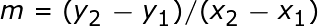# Basic Algebra Practice Problems

Instructor: Sharon Linde
Algebra problems come in lots of different flavors. Check inside for practice on basic algebra problems: one-step, two-step, slope and slope-intercept. Then quiz your understanding to see if you're an algebra ace.

## One-Step Problems

The most basic algebra problems are those that you can do in a single step, also known as one-step problems. I'll be showing all of the steps in the examples, but after you've done a few you may find that you can shorten some of the steps. Here's a word of caution: only do this if you are sure you are ready for it. Skipping steps too early is probably the most common reason for incorrect answers in algebra.

For one-step problems, the basic idea is to get the variable on one side of the equation, and everything else on the other. You do that by doing the opposite operation. Make sure this is done on both sides of the equation, or equal sign! For example, 5x will be divided by 5, y + 2 will have 2 subtracted, etc. Let's do two examples and then some practice problems.

Ex. 1:

-3x = 2

-3x / -3 = 2 / -3 (Divide both sides by -3)

x = -2/3

Ex. 2:

x + 9 = 11

x + 9 - 9 = 11 - 9 (Subtract 9 from both sides)

x = 2

## Two-Step Problems

Two-step problems are a continuation of the one-step problems we just reviewed. The only difference is that you need to choose the order in which to do the steps. Some problems are easier with addition/subtraction first, while others work better with multiplication/division first. The good news is that these types of problems are workable either way; your choices could just make the steps easier. Let's take a look at an example.

2x + 3 = 6

This one looks easier to do subtraction first, unless you prefer to work with fractions.

2x + 3 - 3 = 6 - 3 (Subtract 3 from both sides)

2x = 3 (Combine like terms)

2x/ 2 = 3 / 2 (Divide both sides by 2)

x = 3/2 or 1.5

## Slope Calculation

How do you calculate the slope when you know two points on a line? Use the slope formula, of course!What does that mean? Well, to get the slope, or m, you take the difference in the y values of the two points and divide by the difference in x values of the two points. Be sure that you are consistent in which point you use first. If you accidentally switch them up, you will get a slope with the wrong sign!

What is the slope of a line drawn between the points (-5,5) and (3,-1)?

m= 5 - (-1) / (-5) - 3 = (5 + 1) / (-8) = -6/8 = -3/4 or -0.75

## Slope Intercept Problems

These are some of the most important types of problems you will ever learn in algebra. They can help answer many types of problems in the future, from college courses, to home finances, to job promotions. Using the concepts you'll learn here can help you solve any problem with a linear relationship.

The slope intercept form of a linear equation is typically written as:

y = mx + b

In this equation, m is the slope (which we just learned how to calculate), and b is called the y-intercept. That is just a fancy term to say it intercepts, or crosses, the y axis. Another way to think of it is, 'What does y equal when x is equal to zero?'

Let's do a practice word problem. Many people dislike word problems, but it's been my experience that math problems don't come up in life like they do in a math book. They come up as a real situation you can model with math to help you get answers.

For example, let's say you've been a very good saver, and have \$10,000 in your bank account. However, the company you work for just went out of business. How long will it be before you have \$0 in the bank?

You happen to like nice things, and have a nice house and car. The payments for those items, plus food, entertainment, insurance, and everything else means you are paying \$4,150 a month.

We can now substitute into the slope-intercept equation b = \$10,000 and m = -\$4,150. We want to know what x equals when y = \$0, which is also known as x-intercept.

With that information our equation becomes

To unlock this lesson you must be a Study.com Member.

### Register to view this lesson

Are you a student or a teacher?

### Unlock Your Education

#### See for yourself why 30 million people use Study.com

##### Become a Study.com member and start learning now.
Back
What teachers are saying about Study.com

### Earning College Credit

Did you know… We have over 160 college courses that prepare you to earn credit by exam that is accepted by over 1,500 colleges and universities. You can test out of the first two years of college and save thousands off your degree. Anyone can earn credit-by-exam regardless of age or education level.